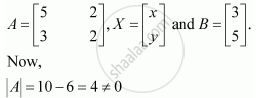Share

# Solve System of Linear Equations, Using Matrix Method. 5x + 2y = 3 3x + 2y = 5 - CBSE (Science) Class 12 - Mathematics

ConceptApplications of Determinants and Matrices

#### Question

Solve system of linear equations, using matrix method.

5x + 2y = 3

3x + 2y = 5

#### Solution

The given system of equations can be written in the form of AX = B, whereThus, A is non-singular. Therefore, its inverse exists.

Is there an error in this question or solution?

#### Video TutorialsVIEW ALL 

Solution Solve System of Linear Equations, Using Matrix Method. 5x + 2y = 3 3x + 2y = 5 Concept: Applications of Determinants and Matrices.
S